Function Repository Resource:

# MovingOrthonormalFrame

Compute the moving orthonormal frame of a surface

Contributed by: Wolfram Staff (original content by Alfred Gray)
 ResourceFunction["MovingOrthonormalFrame"][x,{u,v}] computes the moving orthonormal frame of a parametrized 3D surface x with respect to variables u and v.

## Details and Options

Let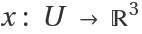be a principal patch with metric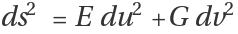. Put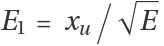and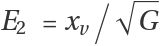. The resulting triple (E1,E2,U), where U denotes the unit normal of x, is called the moving orthonormal frame of x.

## Examples

### Basic Examples (3)

Define the Chebyshev principal patch on the pseudosphere:

 In:=The moving frame associated to a patch x:

 In:=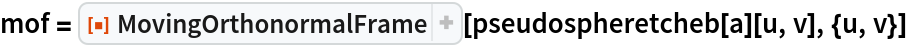Out=In:=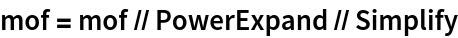Out=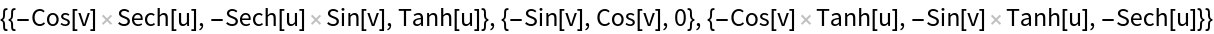Plot the moving frame as u and v are varied:

 In:=Out=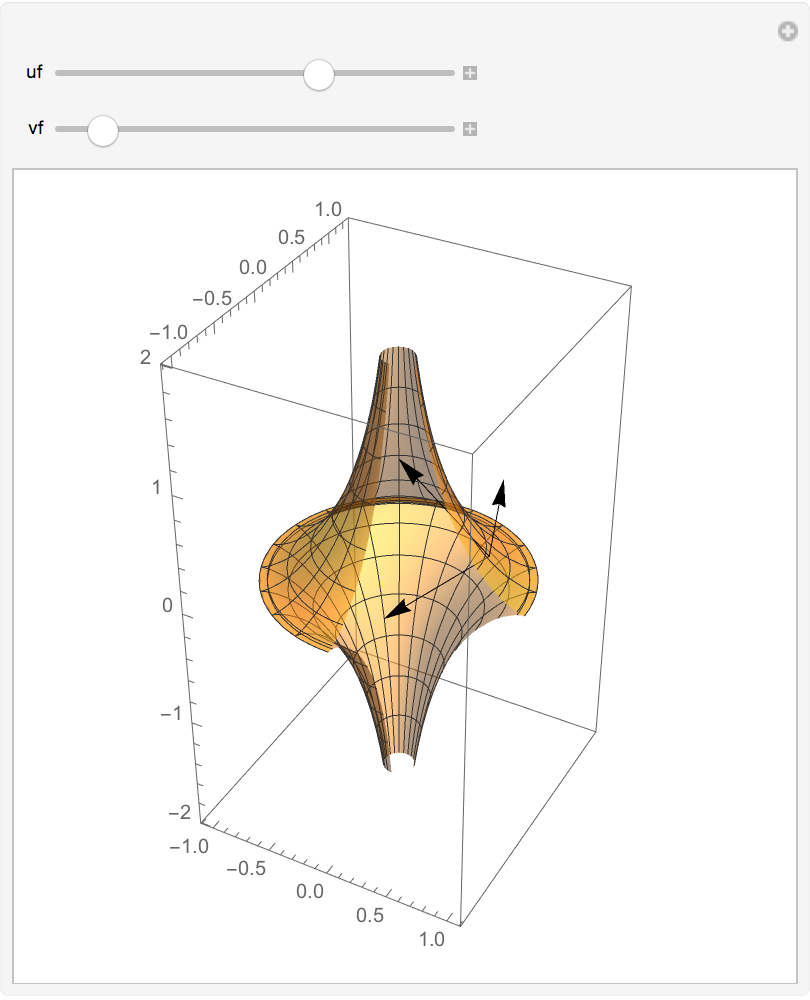Enrique Zeleny

## Version History

• 1.0.0 – 01 December 2020